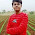Sunday, July 10, 2011

[How to] Solve Brightness problem in ubuntu

If you are facing brightness adjustment problem on you laptop like you can not adjust your laptop brightness using fn keys then try following one...

1. In a terminal window, type in:
Code:
`mousepad ./backlight_d.sh`
(the mousepad application will open if doesnt then install it first)

2. Copy and paste the following into the mousepad application that just opened:
Quote:
 #!/bin/bash old_b=9; declare -i curr_b=240; declare -i target_b=240; while : ; do b=`cat /sys/class/backlight/acpi_video0/brightness`; delay="0.5" if [ \$old_b != \$b ]; then old_b=\$b let "target_b=\$b * 20 + 12" #printf "Target: %10d\n" \$target_b fi hex_b="."; if [ "\$curr_b" -lt "\$target_b" ] ; then let "curr_b=\$curr_b + 2" if [ "\$curr_b" -gt "\$target_b" ] ; then let "curr_b=\$target_b" fi hex_b="-" elif [ "\$curr_b" -gt "\$target_b" ] ; then let "curr_b=\$curr_b - 2" if [ "\$curr_b" -lt "\$target_b" ] ; then let "curr_b=\$target_b" fi hex_b="-" fi if [ \$hex_b != "." ] ; then hex_b=`printf "%02X" \$curr_b` delay="0.005" setpci -s 00:02.0 F4.B=\$hex_b fi sleep \$delay done
3. Save the file:
Code:
`File->Save(save it through closing it)`
4. Back in the terminal window, type in the following:
Code:
`sudo cp ./backlight_d.sh /etc/ && sudo chmod +x /etc/backlight_d.sh`

5. Enter the following into the terminal window:
Code:
`sudo mousepad /etc/rc.local`
(mousepad will open again) and before the exit 0 line, type in the following:
Code:
`nohup /etc/backlight_d.sh &`
so it looks like:
Quote:
 nohup /etc/backlight_d.sh & exit 0
1.